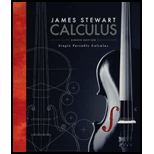# Rewrite by completing the square. (a) x 2 + x + 1 (b) 2 x 2 − 12 x + 11### Single Variable Calculus

8th Edition
James Stewart
Publisher: Cengage Learning
ISBN: 9781305266636### Single Variable Calculus

8th Edition
James Stewart
Publisher: Cengage Learning
ISBN: 9781305266636

Chapter
Section
Textbook Problem
47 views

## Rewrite by completing the square. (a) x2 + x + 1 (b) 2x2 − 12x + 11

Expert Solution

(a)

To determine

To rewrite: The expression x2+x+1 by the method of completing square.

### Explanation of Solution

Consider the expression x2+x+1.

Simplify the above expression as follows,

x2+x+1=x2+2×x×(12)+(12)2(12)2+1=(x+12)214+1

Expert Solution

(b)

To determine

To rewrite: The expression 2x212x+11 by the method of completing square.

### Want to see the full answer?

Check out a sample textbook solution.See solution

### Want to see this answer and more?

Bartleby provides explanations to thousands of textbook problems written by our experts, many with advanced degrees!

See solution

Find more solutions based on key concepts
Show solutions
Rationalize the numerator: x1x1.

Applied Calculus for the Managerial, Life, and Social Sciences: A Brief Approach

Find all possible real solutions of each equation in Exercises 3144. x327=0

Finite Mathematics and Applied Calculus (MindTap Course List)

Sketch the curve and find the area that it encloses. 10. r = 1 sin

Single Variable Calculus: Early Transcendentals

If f(x) 5 for all x [2, 6] then 26f(x)dx. (Choose the best answer.) a) 4 b) 5 c) 20 d) 30

Study Guide for Stewart's Single Variable Calculus: Early Transcendentals, 8th

23. An experiment has been conducted for four treatments with eight blocks. Complete the following analysis of ...

Modern Business Statistics with Microsoft Office Excel (with XLSTAT Education Edition Printed Access Card) (MindTap Course List)

Making Tables and Comparing Functions In Exercises S-1 through S-10, make the table of values. Make a table for...

Functions and Change: A Modeling Approach to College Algebra (MindTap Course List)

In Problems 3740 solve the given boundary-value problem. 38. y 2y + 2y = 2x 2, y(0) = 0, y() =

A First Course in Differential Equations with Modeling Applications (MindTap Course List)